Geometry

# Tangent - Perpendicular to Radius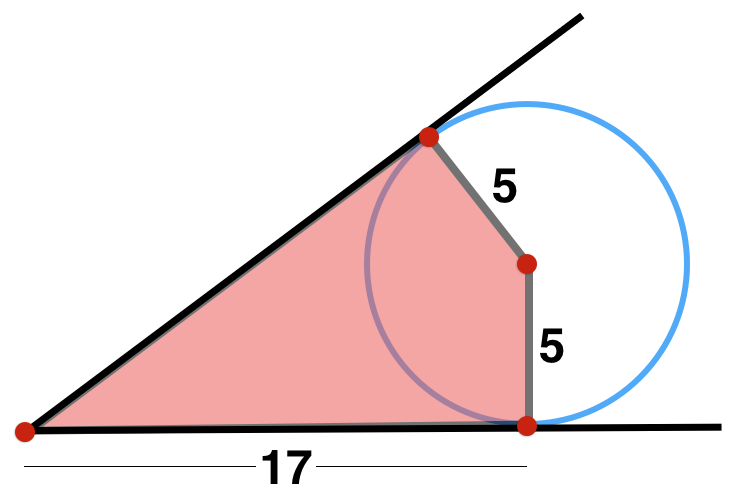What is the area of the shaded quadrilateral shown above?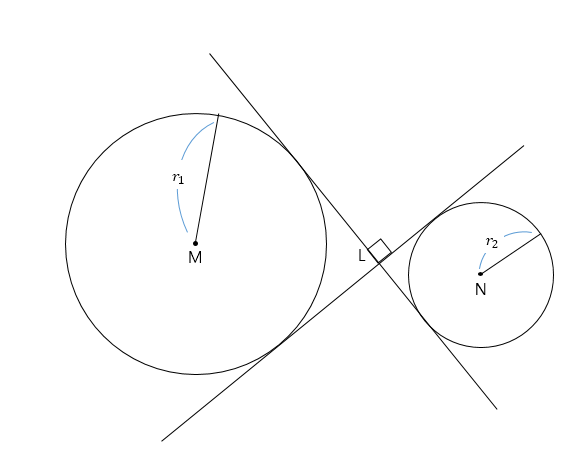Two circles $M$ and $N$ are tangent to two perpendicular lines that intersect at point $L,$ as shown in the above diagram. If the radii of the two circles are $r_{1}=16$ and $r_{2}=8,$ respectively, what is the distance between $M$ and $N?$

Note: The above diagram is not drawn to scale.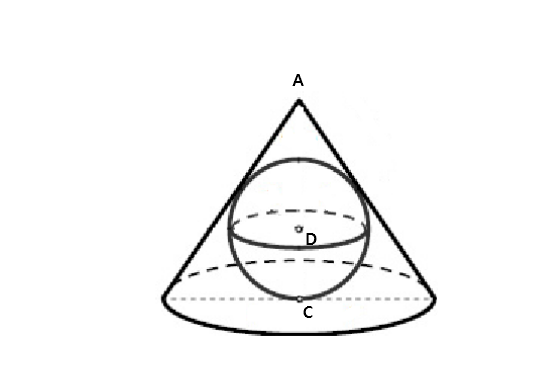In the above diagram, a sphere is inscribed in a right circular cone. If the radius of the sphere is $24$ and the radius of the base circle of the cone is $48,$ what is the height of the cone?

Note: the above diagram is not drawn to the scale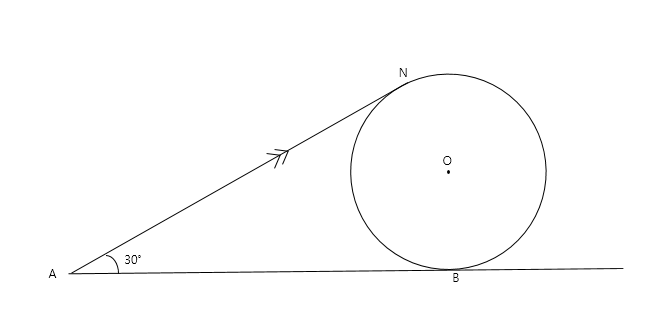$\overline{AN}$ and $\overline{AB}$ are both tangent to circle $O.$ If the radius of the circle is $44$ and $\angle NAB={30}^\circ,$ what is the distance between $N$ and $\overline {AB}?$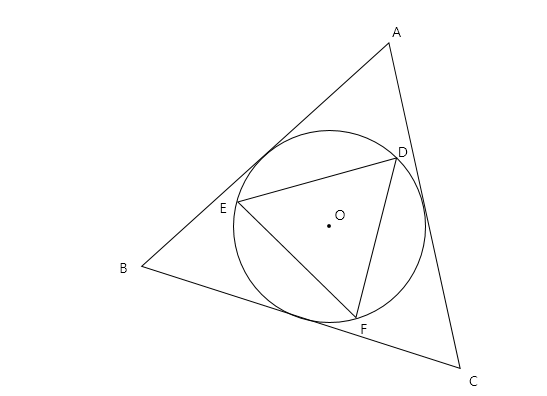In the above diagram, $\triangle ABC$ and $\triangle DEF$ are both equilateral triangles. If the circle in the diagram with center $O$ is the circumscribed circle of $\triangle DEF$ and the inscribed circle in $\triangle ABC,$ what is the ratio $\lvert\overline {AB}\rvert : \lvert\overline {DE}\rvert?$

Note: $\lvert\overline {AB}\rvert$ denotes the length of $\overline {AB}.$

×